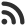Feed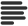Articles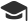Tutorials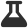CodingLab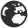CommunityLeaderboardFounder & Programmer at HackersFriend Updated Aug. 13, 2019, 12:25 p.m. ⋅ 76 views

# Merge two Binary search Trees with constant extra space

Problem

You are given 2 Binary Search Trees. Your task is to print elements from both of these tress in sorted order.

Examples

Input

``````
TREE 1:

6
/   \
2     9

TREE 2:

10
/  \
5    15

``````

Output

``2 5 6 9 10 15``

Input

``````TREE 1:

5
/ \
4   7
/
1

TREE 2:

6
/
2
/
0``````

Output

``0 1 2 4 5 6 7``

Solution
We know that, left most element of BST i.e. first element in inorder traversal, is the smalles element of BST. Here we will use this property.

We'll fetch left most element of each BST and compare them, and smallest one and leave the other one as it is in its tree. The element which we just printed will be deleted from its tree.

After this deletion, we'll again get left most elemnt from both tree and print smaller one and delete it from its tree.

We'll keep repeating this process until we completely exhaust one tree.

After anyone of the trees is exhausted, we'll just traverse the other tree in inorder style and print all of its element.

Time complexity of this approach would be  O((X+Y)(h1+h2)), given X and Y are the number of nodes of the each of the trees respectively and, h1 and h2 are the heights of trees respectively.

Space complexity would be O(1)

Implementation

Let's code this approach.

``````#include <bits/stdc++.h>
using namespace std;

// Node of Binary Search Tree
class Node {
public:
int data;
Node* left;
Node* right;
Node(int x)
{
data = x;
left = right = NULL;
}
};

// Inorder traversal of Tree
void inorder(Node* root)
{
if (root != NULL) {
inorder(root->left);
cout << root->data << " ";
inorder(root->right);
}
}

// Required function to print data from both trees in sorted order
void PrintSorted(Node* root1, Node* root2)
{
// Condition to break recursion
if (!root1 && !root2)
return;

// If anyone of the first tree is exhausted
// print the inorder traversal of the second tree
if (!root1) {
inorder(root2);
return;
}

// If second tree is exhausted
// print the inoreder traversal of the first tree
if (!root2) {
inorder(root1);
return;
}

// A temporary to root of first tree
Node* temp1 = root1;

// store the parent of temporary pointer
Node* prev1 = NULL;

// Traverse through the first tree until you reach
// the leftmost element, which is the first element
// of the tree in the inorder traversal.
// This is the least element of the tree
while (temp1->left) {
prev1 = temp1;
temp1 = temp1->left;
}

// Another temporary pointer currently
// pointing to root of second tree
Node* temp2 = root2;

// Previous pointer to store the
// parent of second temporary pointer
Node* prev2 = NULL;

// Traverse through the second tree until you reach
// the leftmost element, which is the first element of
// the tree in inorder traversal.
// This is the least element of the tree.
while (temp2->left) {
prev2 = temp2;
temp2 = temp2->left;
}

// Compare the least current least
// elements of both the tree
if (temp1->data <= temp2->data) {

// If first tree's element is smaller print it
cout << temp1->data << " ";

// If the node has no parent, that
// means this node is the root
if (prev1 == NULL) {

// Simply make the right
// child of the root as new root
PrintSorted(root1->right, root2);
}

// If node has a parent
else {

// As this node is the leftmost node,
// it is certain that it will not have
// a let child so we simply assign this
// node's right pointer, which can be
// either null or not, to its parent's left
// pointer. This statement is
// just doing the task of deleting the node

prev1->left = temp1->right;

// recursively call the PrintSorted
// function with updated tree
PrintSorted(root1, root2);
}
}
else {

cout << temp2->data << " ";

// If the node has no parent, that
// means this node is the root
if (prev2 == NULL) {

// Simply make the right child
// of root as new root
PrintSorted(root1, root2->right);
}

// If node has a parent
else {
prev2->left = temp2->right;

// Recursively call the PrintSorted
// function with updated tree
PrintSorted(root1, root2);
}
}
}

// Main function
int main()
{
Node *root1 = NULL, *root2 = NULL;
root1 = new Node(3);
root1->left = new Node(1);
root1->right = new Node(5);
root2 = new Node(4);
root2->left = new Node(2);
root2->right = new Node(6);

// Print sorted nodes of both trees
PrintSorted(root1, root2);

return 0;
}
``````
``1 2 3 4 5 6``

 arrow_upward UpvoteComment arrow_downward downvote

Events

Nov. 28, 2018, 5:30 p.m.

Python from zero to hero

place Delhi

Aug. 13, 2018, 5:30 p.m.

Python from zero to hero

place Bangalore ( HackersFriend office BTM Layout)